# Topology 1: Counting Shapes

Hey everyone! I’m trying to pen a series of posts of things I love in Math. My self-professed area is Algebraic Topology, but I’ll be talking about ideas from all sorts of areas. In this post, I’m planning to give a brief introduction to the subject, and meet our first invariant (who we’ll see in various guises), the Euler Characteristic.

Algebraic Topology aims to create a tunnel between shapes and algebraic objects (numbers, polynomials, groups, rings, vector spaces, modules and a lot more). These are called invariants¹.

One of the main things we want out of this tunnel is that if two objects have “the same shape”, we want the same number out. This way, the number gives us some information about the shape!

What do we mean by the same shape? If you can continuously deform one shape into another (think of the shape being made of rubber or clay), without cutting, tearing or making holes (we consider these non-continuous), then they have the same shape. We call these shapes homeomorphic. For example, a sphere and a cube have the same shape because I can easily mold one into the other (imagine you inflate the cube like it’s a beach ball). Similarly with a tetrahedron!

Let’s start with polyhedra. These are things like cubes, cuboids, pyramids, bipyramids, octahedra, dodecahedra and so on. Nice clean and flat edges and faces. Let’s investigate our first invariant.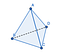Tetrahedron

Here we have an example of a polyhedron, a tetrahedron. Let’s:

Define the Euler Characteristic (χ) as
χ = Number of Vertices (V) — Number of Edges (E) + Number of Faces (F)

In this case we have V = 4 (A, B, C, D), E = 6 (AB, AC, AD, BC, BD, CD) and F = 4 (ABD, ACD, ACB, BCD), giving us χ = 2. Remember this number!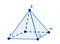Square Pyramid

Let’s try the same calculation with a square pyramid. We have V = 5, E = 8, F = 5 giving χ = 2.

Both of these are “sphere”-like. If these were made of clay or rubber, I could mold one into the other. This hints that in fact for any sphere-like object, you get χ = 2. You can try it yourself with a cube or octahedron! They both give you a χ = 2. Let’s try an example where we have a different χ.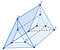Triangular Prism with through-hole

Let’s try something a little different. Here we have a triangular prism where I’ve bored a hole through the middle. The reason for the numerous edges and faces is a little technical: we need everything to be polyhedral and this is surprisingly the easiest one I could come up with. This one takes some work to enumerate so bear with me.

Vertices: 12 (A — L)
Edges: 24 (AB, AD, AE, AI, BC, BE, BK, CD, CF, CK, DF, DG, EF, EJ, FH, GH, GI, GK, HJ, HK, IJ, IL, JL, KL)
Faces: 12 (ABCD, ADFE, BCFE, AIGD, EJHF, BCKL, ABLI, AEJI, BLJE, KLIG, GIJH, JLKH)

I know that was painful, but maybe you can take my word for it. You get a χ = 0, that’s new! This one has a “hole” in the middle that you can’t close through continuous deformation. In fact, this is a doughnut shape if you think about it. I just took a solid sphere-like object (in this case a triangular prism) and bored a hole through it. That’s exactly how you get a doughnut!

Great! Before we continue, two quick questions to ponder.

1. [Well-defined-ness of χ] How do I know that if I choose a different set of V,E,F on the same shape, χ is the same? This would be horrible if not because that means χ isn’t well-defined, you could get many answers for the same shape! It seems like it works though, seem the examples of the filled in square below.

2. [Invariance of χ] I’ve only defined χ for polyhedra! How does this even make sense for smooth surfaces like the sphere and torus? Turns out there’s other ways to define χ and they match what you calculate when you take a homeomorphic polyhedron! See below for an example

These two properties allow us to speak of χ for any smooth surface! Just choose a polyhedron that looks like it, and calculate V-E+F for it. So, we can say

Big Result of this Post: Spheres have χ = 2. Tori have χ = 0.

Here’s the way mathematicians speak. When I say sphere, I actually mean “anything homeomorphic to a sphere”. In topology, (at least initially) we study shapes “up to homeomorphism”. This means that two shapes are the same if they are homeomorphic (which is what we’ve said along).

We now have conclusive evidence that Tori and Spheres are not the same shape, and moreover, cannot be continuously deformed into each other. In the next article, we’ll discuss some applications of this fact. Until then, here’s a small exercise.

Exercise: Connect Sum and Euler Characteristic for all Closed, Connected, Oriented Surfaces²

Given two surfaces M and N, the connect sum of M and N(denoted M#N) is obtained as follows.

Choose a (small) disc on each X and Y, cut them out, and glue X and Y along the boundary circle of the disc.

For example the connect some of the surface with one hole and the surface with two holes gives a three holed doughnut.

1. Show that χ(X#Y) = χ(X) + χ(Y) — 2
Hint: Look at the following diagram. Note that everything outside of the dotted circle stays the same! The only change is inside, and the change occurring is converting 2 discs into one circle.
Try computing χ for 2 discs (hint: it’s homeomorphic to a filled in square!)
Then try computing χ for a circle (hint: it’s homeomorphic to a square!)
How much did χ change by?

2. Therefore, show that connect summing with a torus reduces χ by 2. Similarly show that connect summing with a sphere does not change χ at all.

3. Show that χ of a g-holed Torus³ is 2–2g.

Fun Fact: This invariant completely characterizes (closed, connected, oriented) surfaces. This means that if someone hands you a surface and you just calculate it’s χ, you can say it has the same shape as torus with g holes.
In other words, if you calculate χ for a surface, you can calculate g from the formula above and tell exactly how many holes it has!
Also, the second exercise shows that connect summing with a sphere does not change χ so in fact does not change what the surface is!

Footnotes

¹The tunnels we talked about are called functors. Algebraic topology is the study of functors from topological spaces (shapes) to algebraic categories (numbers, polynomials etc.
²These are technical conditions on the surface. Roughly this is what the words mean. Closed means the surface has no boundary. A sphere has no boundary, whereas a disk has a boundary being the outer circle. Connected means that there is only one piece. Oriented means that the surface has an “inside” and an “outside”.
³The number g in the exercise i.e. the number of holes is called the genus. We have shown that all (closed, connected, oriented) surfaces are characterized completely and uniquely just by their genus.

Technical Aside

So how do we show that two different V, E, F for the same shape give the same χ? The details are a little technical . The idea is that for the two structures on the shape, you can find a common subdivision (for example, by splitting an edge into two edges + a vertex) that have the same number of V, E, F and so the same χ. Notice that splitting an edge below leaves χ unchanged.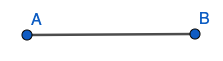Splitting an edge (before) [V = 2, E = 1]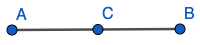Splitting an edge (after) [V = 3, E = 2]

This works for any kind of subdivision, so they always end up at the same final V-E+F!